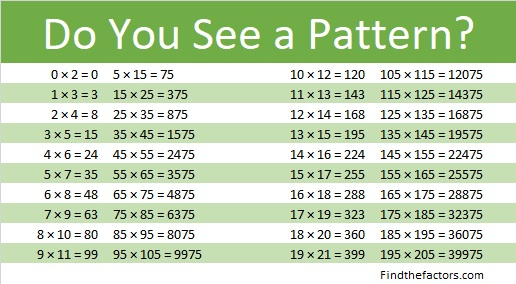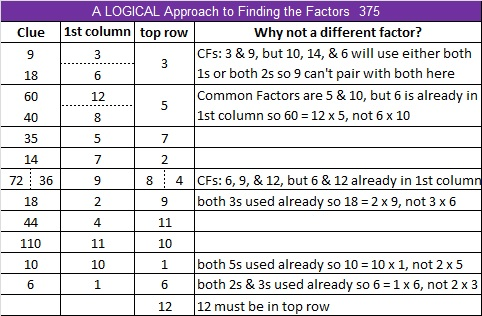# 375 and Level 6

375 is a composite number because its last digit is 5. Scroll down below the puzzle to see its factoring information.Print the puzzles or type the factors on this excel file:12 Factors 2015-01-26

• 375 is a composite number.
• Prime factorization: 375 = 3 x 5 x 5 x 5, which can be written 375 = 3 x (5^3)
• The exponents in the prime factorization are 1 and 3. Adding one to each and multiplying we get (1 + 1)(3 + 1) = 2 x 4 = 8. Therefore 375 has exactly 8 factors.
• Factors of 375: 1, 3, 5, 15, 25, 75, 125, 375
• Factor pairs: 375 = 1 x 375, 3 x 125, 5 x 75, or 15 x 25
• Taking the factor pair with the largest square number factor, we get √375 = (√15)(√25) = 5√15 ≈ 19.365375 is in this cool pattern:This site uses Akismet to reduce spam. Learn how your comment data is processed.